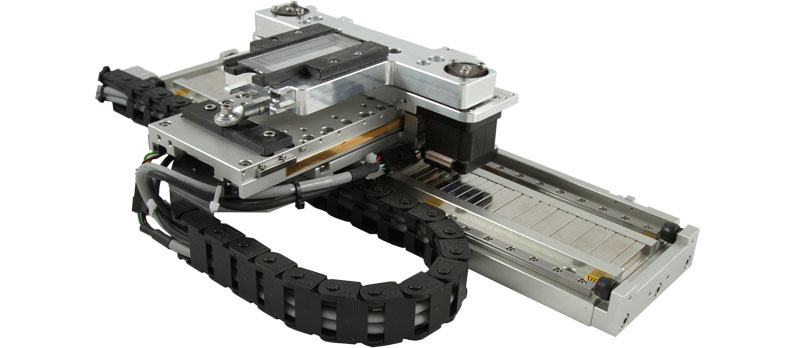# Abbé Error

### Abbé error (pronounced ab-¯a) can be a significant source of error in positioning applications

Named after Ernst Abbé, a noted optical designer, it refers to a linear error caused by the combination of an underlying angular error (typically in the ways which define the motion) and a dimensional offset between the object being measured and the accuracy determining element (typically a leadscrew or encoder). In open loop systems (or closed loop systems employing rotary feedback), the accuracy is nominally determined by the precision of the leadscrew. Similarly, in systems with linear encoders or interferometers, it is that device which determines the accuracy. It is important, however, to recall exactly what information these devices provide: Leadscrews really tell us nothing but the relative position of the nut and screw, and encoders tell us only the position of the read head relative to the glass scale. Extrapolating this to include the position of an item of interest, despite its firm mechanical connection to the nut or encoder read-head, is ill founded.

To illustrate this, consider Figure 6, which shows a single-axis stage with a linear encoder. The stage carries an offset arm which positions a probe over a sample. The apparent distortion in the stage is intentional; it is intended to illustrate, in exaggerated fashion, a stage whose ways have a curvature (in this case, yaw). Someone using this stage, and in possession of appropriate test instruments, would measure an error between the stage position as determined by the encoder read-head, and the actual linear position of the probe.Suppose the curvature is sufficient to produce an angle a’b in Figure 6 of 40 arc-seconds (a’ is drawn parallel to a). If the stage moves forward 300 mm, the probe at the end of the arm will be found to have moved +300.100 mm, resulting in an X-axis error of +100 microns. If the ways were, in fact, curved in a circular arc as shown, there would also be a Y-axis shift of +25 microns. This Y-axis error would be eliminated (while the X-axis error would remain) if the angular error were a purely local property of the ways at the +300 mm location. 100 microns is quite a large error, and Abbé error is accordingly important among the error sources to be considered.

Abbé error is insidious, and can best be countered by assuming the presence of angular error in a system and then working to minimize both the underlying error and its effect, through design optimization and appropriate placement of leadscrews, encoders, etc. The best tool to analyze angular error is the laser interferometer, which, when used with special dual path optics, measures pitch or yaw with 0.025 arc-second (0.125 micro-radian) resolution. We measure roll using a rectangular optical flat, in conjunction with an autocollimator or two capacitance gauges in differential mode.

In the example shown in Figure 6, Abbé error could be lessened by moving the encoder to the left side of the stage. Reducing the arm’s length, or mounting the encoder at the edge of the sample (with the read head connected to the arm) would be more effective. Virtual elimination of Abbé error could be achieved by using a laser interferometer and mounting the moving retroreflector on the probe assembly. Note that the component positions shown in Figure 6 effectively control Abbé error due to pitch error of the stage, since the height of the probe and encoder are roughly equal. While the stage might exhibit a pitch error (rotation around the Y-axis), there is no corresponding vertical (Z-axis) offset needed to produce Abbé error. The third degree of rotational freedom, roll, corresponds in the illustration to the rotation around the axis of motion (X-axis). This would result in the gap between the probe and the sample varying as the stage moved.In general, try to estimate or measure the magnitude of all three possible angular errors (roll, pitch, and yaw) in any given system under actual load bearing conditions. Then, look for any offsets between driving or measuring devices and the point of interest on the load. Calculate the Abbé error, and if it proves unacceptable, optimize the design to reduce either the offset or the underlying angular error. In general, systems built using precision lapped granite and air bearings, which do not extend the load beyond the table base at any point in the travel, are best at minimizing angular errors.

To determine the magnitude of Abbé error, simply multiply the offset by the tangent of the angle. In the example, this was: 500 mm x tan (40 arc-seconds) = 500 x tan (0.011 degrees) = 500 x 0.000194 = 0.100 mm. If the angle is known in radians instead of degrees, the problem is that much easier:

### Abbé error = angle x offset

For example, an angular error of 194 micro-radians, or 0.000194 radians, in conjunction with an offset of 500 mm will result in an Abbé error of 0.000194 x 500 mm = 0.100 mm (not coincidentally, the angle of 194 micro-radians was chosen to match the 40 arc-seconds of the previous example). Finally, a helpful rule of thumb is that the Abbé error will equal about 5 microns per meter of offset and arc-second of angular error; in Imperial units, this amounts to about 5 micro-inches per inch of offset and arc-second of angular error. Once again, 40 arc-seconds x 20 inches x 5 = 4000 micro-inches, or 0.004″. The accompanying chart and figure may prove helpful in determining which offsets produce Abbé error for a given angular error.

wdt_IDAngular ErrorOffset AxisError Axis
1Ox (roll)
Ox
Ox
X
Y
Z
none
Z
Y
2Oy (pitch)
Oy
Oy
X
Y
Z
Z
none
X
3Oz (yaw)
Oz
Oz
X
Y
Z
Y
X
none